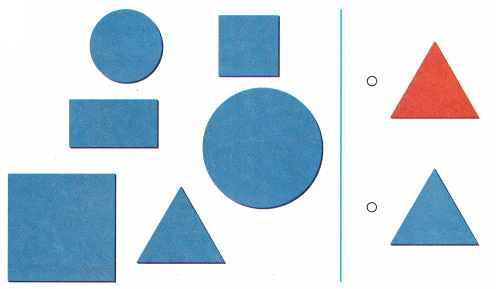# Texas Go Math Kindergarten Lesson 20.1 Answer Key Sort by Color and Size

Refer to our Texas Go Math Kindergarten Answer Key Pdf to score good marks in the exams. Test yourself by practicing the problems from Texas Go Math Kindergarten Lesson 20.1 Answer Key Sort by Color and Size.

## Texas Go Math Kindergarten Lesson 20.1 Answer Key Sort by Color and Size

Essential Question
How can you sort objects by color or by size into two categories?

Explore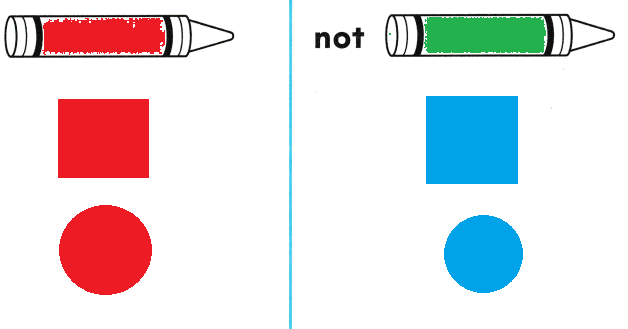Explanation:
In first row the crayon color is red
so, drawn red shapes
In the second row the crayon is green color
so, drawn blue colored shapes.

Directions
Choose a color. Use that color crayon to color the pictures of the crayons at the top of the page. Sort a handful of shapes into a set of that color and a set of shapes that are not that color. Draw and color the shapes to show how you sorted.

Share and Show

Question 1.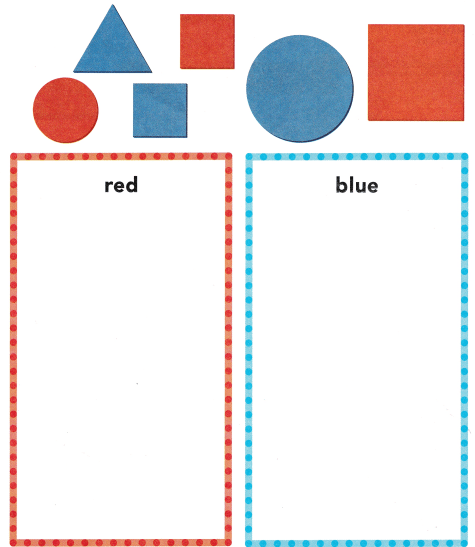Directions 1. Use shapes like those shown at the top of the page. Sort the shapes by color. Draw and color the shapes to show how you sorted them.

Question 2.Explanation:
Shapes are sorted according to the colors
in red color there are rectangle, square, circle.
In the blue box there are triangle, square and circle.

Directions
2. Use shapes like those shown at the top of the page. Sort the shapes by size. Draw and color the shapes to show how you sorted them.

Home Activity

• Give your child several objects, such as buttons. Have your child sort the objects into two sets, by color. Then have your child sort the objects again by size.

Problem Solving

Question 3.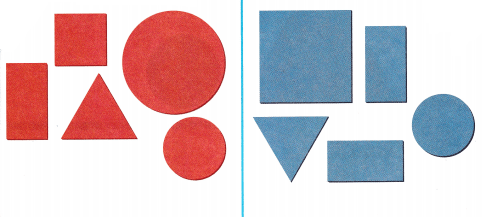Explanation:
The shapes are sorted according to there colors
There are 2 colors red and blue.

Question 4.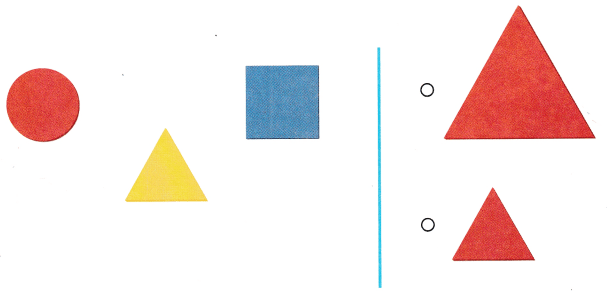Explanation:
The given shapes are in small size
so, marked the small triangle.

Directions
3. How are these shapes sorted? Draw one more shape in each category. 4. Choose the correct answer. Which slope belongs in the set?

### Texas Go Math Kindergarten Lesson 20.1 Homework and Practice

Question 1.Explanation:
Sorted according to the colors
triangle, circle and square in blue color
triangle, circle and square in blue color.

Directions
1. Sort the shapes at the top of the page by color. Draw and color the shapes to show how you sorted them.

Texas Test Prep

Question 2.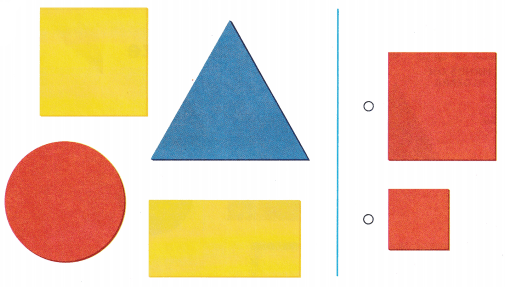Explanation:
A Given shapes are in big size
a red big square is marked.

Question 3.